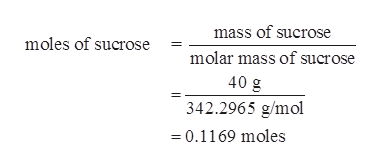How many grams CO2 do you produce when you consume 1 can soda?Given the equation: C6H12O6 + 6O2 -> 6CO2 + 6 H2OGiven 40 grams of sucrose

Question

How many grams CO2 do you produce when you consume 1 can soda?

Given the equation: C6H12O6 + 6O2 -> 6CO2 + 6 H2O

Given 40 grams of sucrose

Step 1

Given,

Mass of sucrose in 1 can soda is 40 grams.

First...help_outlineImage Transcriptionclosemass of sucrose moles of sucrose molar mass of sucrose 40 g 342.2965 g/mol 0.1169 moles fullscreen

Want to see the full answer?

See Solution

Want to see this answer and more?

Our solutions are written by experts, many with advanced degrees, and available 24/7

See Solution
Tagged in

General Chemistry